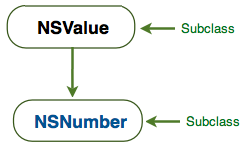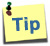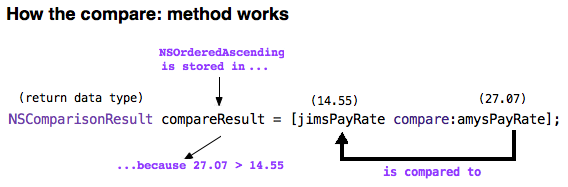The NSNumber class is a subclass of the NSValue class and it is used to box and unbox basic C (int, float, double, char, BOOL) data types because they are not objects. In fact, you can’t use a regular C type anywhere that requires an object. Objects of the NSNumber class are immutable. Once you’ve created and initialize an NSNumber object, you can’t change is value; in other words, an NSNumber object acts exactly like a primitive C variable.

The NSNumber class provides several methods you can use to box and unbox C values and you will learn how to use a few of them in here.

 To practice source code presented in this lesson, you should download and unzip this file.Launch the project (CodePractice.xcodeproj) and enter code in the viewController.m file’s buttonTapped method, then click the “Click Me” button to execute the method’s code.

### The numberWithFloat: Method

You can use an instance of the NSNumber class where an object is required. For example, here’s how you box a C floating-point number, using the numberWithFloat method.

```// Create an NSNumber object, box the C floating-point number, then assign it to the NSNumber object
NSNumber *priceObject = [NSNumber numberWithFloat:240.44];
```

Now that you’ve boxed a C floating-point number, you can add it in an Objective-C’s object; such as an NSDictionary object.

```NSDictionary *bookObject = [NSDictionary dictionaryWithObjectsAndKeys:priceObject,@"kPrice",
@"Charlaine Harris",@"kauthor",
nil];

NSLog(@"%@",bookObject);
```

Output
{
kPrice = "240.44";
kTitle = "Living Dead in Dallas";
kauthor = "Charlaine Harris";
}

### The numberWithInt: Method

Code shown below demonstrates how to use the numberWithInt: method to box a primitive C int variable.

```// Declare and initialize an integer variable
int age = 17;

// Box the integer variable and store it in an object variable
NSNumber *boxedAge = [NSNumber numberWithInt: age];

// Display the boxedAge object variable in the TextView control
self.outputBox.text = [NSString stringWithFormat:
@"age = %@",boxedAge];
```

Output
age = 17

#### Other Common Boxing Methods

This table provide other common methods of the NSNumber class you can use to box C values.

Method Example of Use
numberWithBool NSNumber *canVote = [NSNumber numberWithBool:YES];
numberWithChar NSNumber *charObject = [NSNumber numberWithChar:’A’];
numberWithLong NSNumber *aLongNumber = [NSNumber numberWithLong: 674407591];
numberWithShort NSNumber *aShortNumber = [NSNumber numberWithShort: 1067];
numberWithDouble NSNumber *salePrice = [NSNumber numberWithDouble:3289.97];

Instead of using above methods to box C values, you can use these NSNumber literals.

```NSNumber *canVote = @YES;
NSNumber *charObject = @'A';
NSNumber *boxedAge = @17;
NSNumber *aLongNumber = @674407591;
NSNumber *productPrice = @499.95;
NSNumber *salePrice = @3289.97;
```

You can box an expression using the literal @() syntax; thus turning a basic arithmetic calculation into an NSNumber objet, as this code demonstrates.

```float bookPrice = 14.95;
float taxRate = 0.15;

// Box the arithmetic expression before assigning it to the result variable
NSNumber *result = @(bookPrice * taxRate);
```

### The floatValue Method

Unboxing an NSNumber object is the opposite of boxing a C value. When you unbox an NSNumber object, you are basically converting it from an Objective-C object to a C variable. For example, the floatValue method is used for unboxing a boxed float value. For example, to unbox the result NSNumber object shown in above code, you’d have to use the floatValue method. You then display the unboxed variable in the TextView control, as shown below:

```// Unbox the NSNumber object, assign it to an float variable
float unboxedResult = [result floatValue];

// Display the unboxed object in the UITextView control
self.outputBox.text = [NSString stringWithFormat:@"Sales tax amount is %.2f",unboxedResult];
```

You could alternatively do the unboxing and assignment in a single statement like this:

```self.outputBox.text = [NSString stringWithFormat:@"Sales tax amount is %.2f", [result floatValue]];
```

Output

By the way, the method used to unbox a NSNumber object must match the method used to box it. An attempt, for example, to unbox the boxedResult object, using the intValue: method, will provide unexpected result. That’s because the boxedResult object was boxed using the literal syntax, @().

#### The stringValue Method

This method is used to convert a boxed C value to an NSString object. Code below shows how to use  the stringValue method.

```// Box a double value (29.95)
NSNumber *money = [NSNumber numberWithDouble:29.95];

// Convert the boxed double value to a string object
NSString *stringMoney = [money stringValue];

// Display the string object in the UITextView control
self.outputBox.text = [@"The stringMoney object contain this value: " stringByAppendingString:stringMoney];
```

Output

#### Other Common Unboxing Methods

This table provide other common methods of the NSNumber class you can use to unbox C values.

Method
boolValue
charValue
intValue
longValue
shortValue
doubleValue
decimalValue

#### The isEqualToNumber: Method

The isEqualToNumber: method is used to compare two NSNumber objects to see if they are equal. If they are, the method returns the Boolean value YES otherwise NO. Code below demonstrates how to use the isEqualToNumber: method. The value returned by the method is used in an if statement to display a message in the TextView control.

```// Instantiate and initialize two NSNumber objects
NSNumber *jimsPayRate = [NSNumber numberWithDouble:14.55];
NSNumber *amysPayRate = [NSNumber numberWithDouble:27.07];

// Check to see if both object's values are equal, store result of comparison in a variable
NSUInteger payRatesAreTheSame = [jimsPayRate isEqualToNumber: amysPayRate];

// Check the variable value
if (payRatesAreTheSame)
self.outputBox.text = @"Both pay rates are equal.";
else
self.outputBox.text = @"Pay rates are not equal.";
```

OutputWhat is the difference between an NSInteger and an NSNumber. Well, an NSInteger is simply a C integer, where as an NSNumber is an object.

#### Code Note

When this statement is executed,

```NSUInteger payRatesAreTheSame = [jimsPayRate isEqualToNumber: amysPayRate];
```

the number zero is assigned to the payRateAreTheSame variable because the value assigned to jimsPayRate and amysPayRate are not equal. If they were, the number 1 would have been assigned to the payRatesAreTheSame variable.

### The Compare: Method

This is a method of the NSNumber class and it is used for comparing values assigned to two NSNumber objects. The compare: method returns the result of the comparison in the form of an NSComparisonResult enumerator. Here is the syntax of the compare: method.

```NSComparisonResult compareResult = [NSNumberObjectOne compare: NSNumberObjectTwo];
```

The compare: method works by comparing the NSNumberObjectTwo to the NSNumberObjectOne, returns the result of the comparison in the compareResult variable. Possible settings assigned to the enumerator variable, compareResult are listed below:

NSOrderedSame
If both objects’ numbers are equal, NSOrderedSame is the value stored in the enumerator variable.

NSOrderedAscending
If the second object’s number (located to the right of the compare: method), is greater than the first object’s number, NSOrderedAscending is stored in the enumerator variable.

NSOrderedDescending
If the second object’s number (located to the right of the compare: method), is less than the first object’s number, NSOrderedDescending is the value stored in the enumerator variable.Code below shows how to use the compare: method.

```// Declare and initialize two NSNumber objects
NSNumber *jimsPayRate = [NSNumber numberWithDouble:14.55];
NSNumber *amysPayRate = [NSNumber numberWithDouble:27.07];

// Compare amysPayRate to jimsPayRate
NSComparisonResult compareResult = [jimsPayRate compare:amysPayRate];

// Check compareResult and display an appropriate message
if (compareResult == NSOrderedSame)
outputBox.text = @"Pay rates are equal.";
else if (compareResult == NSOrderedAscending)
outputBox.text = @"Amy's pay rate is greater than Jim's pay rate.";
else if (compareResult == NSOrderedDescending)
outputBox.text = @"Amy's pay rate is less than Jim's pay rate.";
```

Output

Code Note

Code in the second else if statement is executed because, 27.07 is greater than 14.55. The way to figure out which if statement code is executed, say and answer this out loud:

Is objectTwo’s number (Amy’s pay rate) < objectOne’s number (Jim’s pay rate)?

I hope above technique makes sense to you. If not, take a look again at above diagram of how the compare: method works.